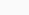# Electricity tariffs in Spain. How do they work?

## Electricity tariffs in Spain. How do they work?

Electricity tariffs in Spain is not charged the same to an individual as to an industry, access rates are defined in Royal Decree 1164/2001. The conditions for selecting one or the other vary depending on the voltage level and the level of contracted power.

#### 2.0 Rates

• Low voltage.
• Power values: up to 10 kW of power can be contracted. Until October 2018, the contractable power values ​​were established and it was not possible to select a different option from the list. From the Royal Decree Law 15/2018, for remote management meters it is possible to contract any power value with a decimal (0.1 kW).
• Power measurement and control: for these types of rates, the contracted power was limited with the Power Control Switch (ICP). This switch was in charge of cutting the electrical supply when the power demanded exceeds the contracted limit. Currently, with remote management meters, the ICP is not necessary, the meters themselves are capable of measuring the power demanded. Every 15 minutes the meter checks if the requested power value exceeds the contracted power, if so, it cuts the supply. It also has automatic reactivating programs.
• Power billing: the contracted power is billed directly at the established fixed price.

#### Rates 2.1

They are identical to 2.0 tariffs, with the difference that the power to be contracted must be between 10 and 15 kW.

#### Rate 3.0

• Low voltage.
• Power values: three billing periods are differentiated, each with an independent contracted power. The limitation is that at least one of them has to have more than 15 kW contracted. At this rate, any power value can be contracted without decimal limits.
• Power measurement and control: the meter’s maximeter is used to measure power. The maximum power value for each period is recorded within the billing time.
• Power billing: knowing the readings of the maximeters and the contracted powers, the power to be billed is calculated. In summary, it would be charged, for each period, as follows:
• If the maximeter is below 85% of the contracted power, 85% of the contracted is billed.
• If the maximeter is between 85% and 105% of the contracted amount, the power registered by the maximeter is billed.

#### Rate 3.1

• High Voltage, up to 30 kV.
• Power values: an upper limit of contractable power of 450 kW is established, without a lower limit. Furthermore, the three periods must have increasingly contracted powers (P3> P2> P1) or be the same. Any power value can be contracted without a decimal limit.
• Measurement and control, as well as billing, is done the same as with rate 3.0.

#### Rates 6.X

• High voltage.
• Power values: for voltages up to 30 kV, at least one of the periods must have a contracted power greater than 450 kW. In the event that the voltage is higher, it is possible to contract any power value. 6 periods of powers are established, in an increasing way (P6> P5> P4> P3> P2> P1).
• Power measurement and control: the power is measured more specifically, recording all the maximeters every 15 minutes and for each period.
• Power billing: the power to be billed coincides with the contracted power for each of the periods. If powers higher than those contracted for each period are recorded, a billing term is applied for these excesses. This term has a somewhat more complex formula to explain it in a few words. If you are interested in hiring our energy advisory services in this area, we invite you to contact us for more details.

Fundamentally, the part corresponding to power has been discussed, since energy corresponds to the free market. In energy, the same periods are generally established as for power and what is consumed is paid at the agreed price. In the case of tariffs 2.0 and 2.1, the Law regulates 3 different types of tariffs for energy. We will talk about this later in future posts.